## 3.10Upwind scheme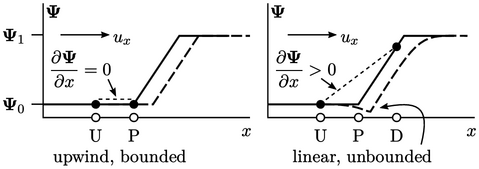The upwind scheme represents the face value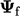by the value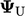in the cell upwind of the face. The advantage of upwind is that it can guarantee boundedness of a ﬁeld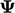. We can demonstrate this point by revisiting the 1D Eq. (2.32 ) in Sec. 2.9 :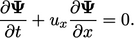In the graphic above, we track the translation of a proﬁle ofby equating changes inin time to the local gradient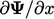. If we apply upwind to calculate the change at point P, the gradient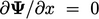and no change inis correctly calculated (left).

However, linear diﬀerencing between upwind and downwind values results in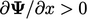, so predicts a decrease in the value at P (right). The solution produces a solution with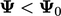, so is unbounded.

Boundedness of the conservative form of advection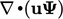is only guaranteed when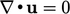, as discussed in Sec. 2.9 . In 1D, the conservative form moves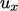inside the derivative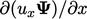. That gradient is only zero with upwind when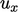is uniform, i.e. the 1D equivalent to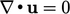.

### Diﬀusion of upwind

The upwind scheme is highly diﬀusive which can result in poor accuracy. Its diﬀusive nature can be explained by considering the following Taylor’s series expansion: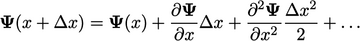(3.9)
In our 1D example, the upwind scheme calculates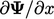using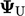and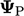at locations U and P, separated by distance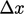. Relating the upwind calculation to Eq. (3.9 ) gives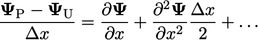(3.10)
In other words, the upwind discretisation represents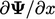but also the second derivative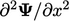(and higher derivatives).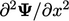is equivalent to a Laplacian, described in Sec. 2.14 , which diﬀuses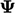with a diﬀusivity proportional to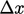.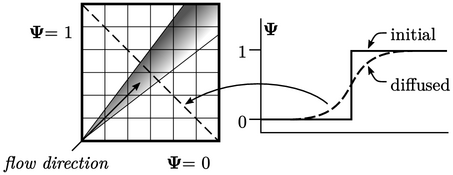The upwind scheme is particularly diﬀusive when the ﬂow direction is not aligned with the cells of a mesh. In the 2D box of cells above,is advected at a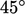angle, beginning with an abrupt step change from= 1 and= 0 between the left and lower boundaries. The step rapidly diﬀuses along the direction of travel as shown in graph (right) and shaded area (left).

Notes on CFD: General Principles - 3.10 Upwind scheme